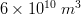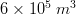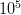# Boxes

## Overview

A box file is used to define an area, which can be 2D or 3D. These areas can be used to initialize properties fields or integrate values in time and space inside and between boxes, allowing computing global and zonal budgets for almost every modeled variables. Module BoxDif is the module responsible for handling and interpreting the boxes files.

## Boxes file

Boxes files can be automatically generated by MOHID GIS based on a bathymetry file and on a polygon file.

A box file requires the definition polygons. These polygons are defined by vertices indicated by the grid cell [i,j] indexes. Thus, a box file is always specific of a certain grid file. Please note that in polygon files, used in MOHID GIS, the polygon vertices are defined by real coordinates [x,y].

In a box file, several boxes can be defined. An ID number is given to each box, based on the order in which the box file is read. Box 1 is the first block in the file, Box 2 the second and so on. By default a Box 0 (zero) is always created, corresponding to the box containing all the grid points that were not included inside the boxes. This way if box integration is requested and no boxes are defined, the output will be the integration of the entire domain.

When used to integrate values, the correspondent outputs are written in Time Series format. Thus, in the box file needs information on the time step to write results, which is given by keyword:

DT_OUTPUT_TIME                :  3600


As a safety feature, a Grid Data file can be written in the beginning of a simulation, where in to each grid cell, the index of the corresponding box is written. This can be done by defining keyword:

WRITE_BOXES                   : 1


and then the name of the output file to be written:

OUTPUT_FILE                   : ..\GeneralData\Boxes\BoxesFileInGridDataFormat.dat


by default this file is written on the exe folder.

This sets the time-step to compute only every 300 seconds (default is 300s).

DT_BOXES                       : 300


### Sample

This example shows the definition of a box with 4 vertices. By default Module BoxDif automatically introduces an extra vertice at the end of the list which is equal to the first vertex, thus insuring the polygon is closed.

#### 2D box file

DT_OUTPUT_TIME                : 3600
WRITE_BOXES                   : 0
DT_BOXES                      : 300

<beginpolygon>
<<beginvertix>>
44 39
33 77
71 88
82 49
<<endvertix>>
<endpolygon>


#### 3D box file

This example shows the definition of two boxes, defined by the same polygon, but which integrate in the vertical direction, from layer 1 to 5, and from layer 6 to 10.

DT_OUTPUT_TIME                : 3600
WRITE_BOXES                   : 0
DT_BOXES                      : 300

<beginpolygon>
<<beginvertix>>
44 39
33 77
71 88
82 49
<<endvertix>>
<<beginverticallayer>>
1  5
6  10
<<endverticallayer>>
<endpolygon>


## Initialization Boxes

Boxes can be used to initialize a waterproperty. The waterproperty concentration for each box must be given ordered along the box id starting from Box 1 to the last Box. Box 0 is set accordingly to the default value of the waterproperty.

### Module WaterProperties

Boxes values are set for Box 1 and Box 2 (3D sample). Default value will be set in Box 0.

<beginproperty>
...
INITIALIZATION_METHOD     : BOXES
FILENAME                  : ..\General Data\Initial Conditions\Boxes.dat
BOXES_VALUES              : 15.5 25.3
DEFAULTVALUE              : 36.
...
<endproperty>


### Module Lagrangian

Boxes can be used to initialize a polygon with lagrangian tracers as opposed to a pointwise discharge. For each lagrangian origin, the box number (one box per origin and ID of lagrangian tracers) and the initial volume of one lagrangian particle must be given. The lagrangian box initial emission is such that the total volume of lagrangian particles is equal to the total volume of the box. Thus, if the box has a volume of$6\times 10^{10}\; m^3$ and the lagrangian initial volume (set in keyword BOXVOLINIC) is$6\times 10^5\; m^3$, then a total of$10^5$ lagrangian particles will be initialy distributed evenly inside the box. The higher the number the of lagrangian particles and the longer the time of computation in a linear proportionality so the BOXVOLINIC is a critical parameter to determine the duration of the simulation.

PARTIC_BOX                : ..\General Data\Initial Conditions\Boxes.dat
...
<BeginOrigin>
...
EMISSION_SPATIAL          : Box
EMISSION_TEMPORAL         : Instantaneous
BOXVOLINIC                : 600000
BOX_NUMBER                : 1
...
<EndOrigin>


## Monitoring Boxes

Regarding water quality parameters such as nutrients, there are 2 output files created by the model: a BXF and a BXM file. The first shows the instantaneous fluxes between the existing boxes, and the second shows the instantaneous mass of the property simulated (ex:ammonia, nitrate, etc) in the entire box.

The values for the instantaneous fluxes are g/s (for ammonia : gN/s) and for the instantaneous mass, in grams.

As for water fluxes the units are, for the BXF file, in m3/s, and for the BXM file in m3.

Regarding the lagrangian particules, there is one output file per box: a LBM file. This file contains the total volume of lagrangian tracers per origin at each time instant, one origin per column.

### Module Hydrodynamic

BOXFLUXES                 : ..\General Data\Initial Conditions\Boxes.dat


### Module WaterProperties

BOXFLUXES                 : ..\General Data\Initial Conditions\Boxes.dat
...
<beginproperty>
...
BOX_TIME_SERIE            : 1
...
<endproperty>


### Module Lagrangian

MONITOR_BOX               : ..\General Data\Initial Conditions\Boxes.dat
...
<BeginOrigin>
...
BOX_TIME_SERIE            : 1
...
<EndOrigin>